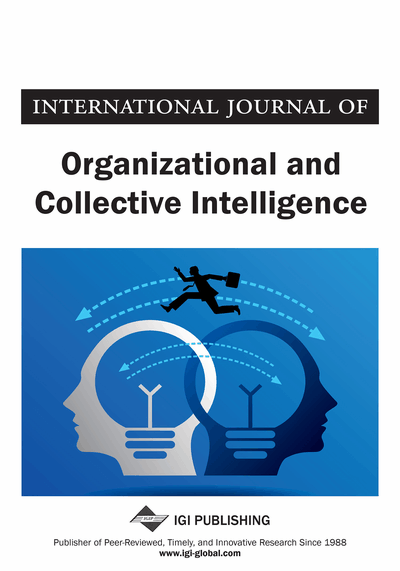Learning Transformations with Complex-Valued Neurocomputing

Tohru Nitta (National Institute of Advanced Industrial Science and Technology (AIST), Tsukuba, Japan)
DOI: 10.4018/joci.2012040103
Available
\$37.50
No Current Special Offers

Abstract

The ability of the 1-n-1 complex-valued neural network to learn 2D affine transformations has been applied to the estimation of optical flows and the generation of fractal images. The complex-valued neural network has the adaptability and the generalization ability as inherent nature. This is the most different point between the ability of the 1-n-1 complex-valued neural network to learn 2D affine transformations and the standard techniques for 2D affine transformations such as the Fourier descriptor. It is important to clarify the properties of complex-valued neural networks in order to accelerate its practical applications more and more. In this paper, first, the generalization ability of the 1-n-1 complex-valued neural network which has learned complicated rotations on a 2D plane is examined experimentally and analytically. Next, the behavior of the 1-n-1 complex-valued neural network that has learned a transformation on the Steiner circles is demonstrated, and the relationship the values of the complex-valued weights after training and a linear transformation related to the Steiner circles is clarified via computer simulations. Furthermore, the relationship the weight values of the 1-n-1 complex-valued neural network learned 2D affine transformations and the learning patterns used is elucidated. These research results make it possible to solve complicated problems more simply and efficiently with 1-n-1 complex-valued neural networks. As a matter of fact, an application of the 1-n-1 type complex-valued neural network to an associative memory is presented.
Article Preview
Top

Neural Network Model

A brief overview of neural networks is given. In the early 1940s, the pioneers of the field, McCulloch and Pitts, proposed a computational model based on a simple neuron-like element (McCulloch & Pitts, 1943). Since then, various types of neurons and neural networks have been developed independently of their direct similarity to biological neural networks. They can now be considered as a powerful branch of present science and technology.

Neurons are the atoms of neural computation. Out of those simple computational neurons all neural networks are build up. An illustration of a (real-valued) neuron is given in Figure 1. The activity of neuronis defined as:

(1)whereis the real-valued weight connecting neuron n and m,is the real-valued input signal from neuron, andis the real-valued threshold value of neuron. Then, the output of the neuron is given by. Although several types of activation functionscan be used, the most commonly used are the sigmoidal function and the hyperbolic tangent function.

Complete Article List

Search this Journal:
Reset
Open Access Articles
Volume 12: 4 Issues (2022): 2 Released, 2 Forthcoming
Volume 11: 4 Issues (2021)
Volume 10: 4 Issues (2020)
Volume 9: 4 Issues (2019)
Volume 8: 4 Issues (2018)
Volume 7: 4 Issues (2017)
Volume 6: 4 Issues (2016)
Volume 5: 4 Issues (2015)
Volume 4: 4 Issues (2014)
Volume 3: 4 Issues (2012)
Volume 2: 4 Issues (2011)
Volume 1: 4 Issues (2010)
View Complete Journal Contents Listing